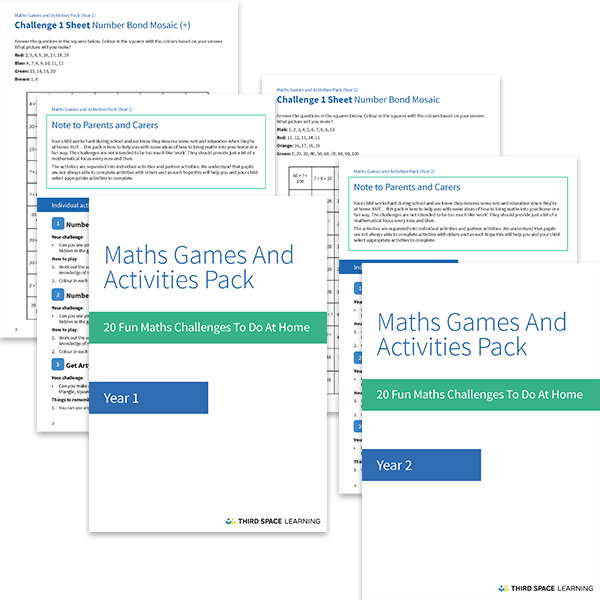Teaching support from the UK’s largest provider of in-school maths tuitionone to one lessonsschools supported

Deepen mathematical understanding

396 mastery-aligned teaching units designed to plug individual gaps and delivered by specialist tutors

See it in action

Hundreds of FREE online maths resources!

Daily activities, ready-to-go lesson slides, SATs revision packs, video CPD and more!Explore all resources
Dictionary & Wiki

Deepen mathematical understanding

396 mastery-aligned teaching units designed to plug individual gaps and delivered by specialist tutors

See it in action

# What Is A Number Sentence: Explained For Primary Parents And Kids!

In this post we will be answering the question “what is a number sentence?” and running through everything you need to know about this particular part of primary maths. We’ve also got a number of number sentence questions you can use to test out your child’s skills, so make sure you scroll to the bottom of the post!

### What is a number sentence?

A number sentence is a combination of numbers and mathematical operations that children are often required to solve.

Examples of number sentences include:

32 + 57 = ?

5 x 6 = 10 x ?

103 + ? = 350

They will usually comprise of addition, subtraction, multiplication or division – or a combination of all four!

Remember – You may consider the above simply as “sums”, but referring to them as this can be confusing for children because the word “sum” should only be used when discussing addition.KS1 Maths Games and Activities Pack

A FREE resource including 20 home learning maths activities and games for years 1 and 2 children to complete on their own or with a partner.Download Free Now!

### When will my child learn about number sentences?

In the National Curriculum, number sentences are referred to as ‘mathematical statements’ – these are introduced from Year 1, where pupils read, write and interpret mathematical statements involving addition (+), subtraction (–) and equals (=) signs.

Number sentences build on what children will have already learnt about number bonds.

Year 2 pupils calculate mathematical statements for multiplication and division within the multiplication tables and write them using the multiplication (×), division (÷) and equals (=) signs.

Year 3 pupils write and calculate mathematical statements for multiplication and division using the multiplication tables that they know, including for two-digit numbers times one-digit numbers, using mental and progressing to formal written methods.

These pupils continue to practise their mental recall of multiplication tables when they are calculating mathematical statements in order to improve fluency.

Year 4 pupils write statements about the equality of expressions (for example, use the distributive law 39 × 7 = 30 × 7 + 9 × 7 and associative law (2 × 3) × 4 = 2 × (3 × 4).

Year 5 pupils are expected to understand the terms factor, multiple and prime, square and cube numbers and use them to construct equivalence statements (for example, 4 x 35 = 2 x 2 x 35; 3 x 270 = 3 x 3 x 9 x 10 = 81 x 10).

They should also recognise mixed numbers and improper fractions and convert from one form to the other and write mathematical statements > 1 as a mixed number [for example, 2/5 + 4/5 = 6/5 = 1 and 1/5].

Year 6 pupils continue to use all the multiplication tables to calculate mathematical statements in order to maintain their fluency.

Wondering about how to explain other key maths vocabulary to your children? Check out our Primary Maths Dictionary, or try these other maths terms:

### Number sentence practice questions

1) Complete the number sentences.

340 ÷ 7 = ____  remainder ____

____÷ 3 = 295 remainder 2

2) Here is a number sentence.

____ + 27 > 85

Circle all the numbers below that make the number sentence correct.

30           40           50           60           70

3) Write in the missing number.

Online 1-to-1 maths lessons trusted by schools and teachers
Every week Third Space Learning’s maths specialist tutors support thousands of primary school children with weekly online 1-to-1 lessons and maths interventions. Since 2013 we’ve helped over 150,000 children become more confident, able mathematicians. Learn more or request a personalised quote to speak to us about your needs and how we can help.

Primary school tuition targeted to the needs of each child and closely following the National Curriculum.##### Ellie Williams
Third Space Learning
Content Team
With a love for all things KS2 maths, Ellie is a part of the content team that helps all of the Third Space Learning blogs and resources reach teachers!

Related Articles

x#### KS1 Maths Games and Activities Pack

A FREE resource including 20 home learning maths activities and games for years 1 and 2 children to complete on their own or with a partner.

#### KS1 Maths Games and Activities PackA FREE resource including 20 home learning maths activities and games for years 1 and 2 children to complete on their own or with a partner.